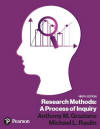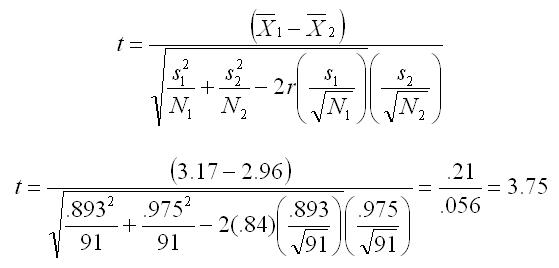﻿ Manual Computation of the Correlated Samples t-testGraziano & Raulin
Research Methods (9th edition)

## Correlated Samples t-Test

The correlated samples t-test, also called the direct difference t-test, compares scores from two conditions in a within-subjects design or two groups in a matched-subjects design. The formula shown here uses values that you have already learned to compute: Means, Variances and Standard Deviations, and a product-moment correlation

In this example, anxiety ratings were made before and after a brief treatment session. The ratings range from 1 (no anxiety) to 5 (extreme anxiety). Pretest and posttest ratings of anxiety are made on 91 subjects. Listed here are the summary statistics computed from the raw data.

 Group 1 Group 2 Mean 3.17 2.96 Standard Deviation 0.893 0.975 Sample Size N = 91 Correlation r = 0.84

Use the formula below to compute the value of t.Look up the critical value of t (df = number of pairs - 1 = 91 - 1 = 90). With an alpha of 0.05, the critical value of t is between 1.98 and 2.00. Because the observed t (3.75) exceeds the critical value, we reject the null hypothesis and conclude that the treatment produced a significant reduction in anxiety.